# Introduction to the pycox package¶

In this notebook we introduce the use of pycox through an example dataset. We illustrate the procedure with the LogisticHazard method (paper_link), but we this can easily be replaced by for example PMF, MTLR or DeepHitSingle.

In the following we will:

• Load the METABRIC survival dataset.
• Process the event labels so the they work with our methods.
• Create a PyTorch neural network.
• Fit the model.
• Evaluate the predictive performance using the concordance, Brier score, and negative binomial log-likelihood.

While some knowledge of the PyTorch framework is preferable, it is not required for the use of simple neural networks. For building more advanced network architectures, however, we would recommend looking at the PyTorch tutorials.

## Imports¶

You need sklearn-pandas which can be installed by uncommenting the following block

In :
# ! pip install sklearn-pandas

In :
import numpy as np
import matplotlib.pyplot as plt

# For preprocessing
from sklearn.preprocessing import StandardScaler
from sklearn_pandas import DataFrameMapper


pycox is built on top of PyTorch and torchtuples, where the latter is just a simple way of training neural nets with less boilerplate code.

In :
import torch # For building the networks
import torchtuples as tt # Some useful functions


We import the metabric dataset, the LogisticHazard method (paper_link) also known as Nnet-survival, and EvalSurv which simplifies the evaluation procedure at the end.

You can alternatively replace LogisticHazard with, for example, PMF or DeepHitSingle, which should both work in this notebook.

In :
from pycox.datasets import metabric
from pycox.models import LogisticHazard
# from pycox.models import PMF
# from pycox.models import DeepHitSingle
from pycox.evaluation import EvalSurv

In :
# We also set some seeds to make this reproducable.
# Note that on gpu, there is still some randomness.
np.random.seed(1234)
_ = torch.manual_seed(123)


## Dataset¶

We load the METABRIC data set as a pandas DataFrame and split the data in in train, test and validation.

The duration column gives the observed times and the event column contains indicators of whether the observation is an event (1) or a censored observation (0).

In :
df_train = metabric.read_df()
df_test = df_train.sample(frac=0.2)
df_train = df_train.drop(df_test.index)
df_val = df_train.sample(frac=0.2)
df_train = df_train.drop(df_val.index)

In :
df_train.head()

Out:
x0 x1 x2 x3 x4 x5 x6 x7 x8 duration event
0 5.603834 7.811392 10.797988 5.967607 1.0 1.0 0.0 1.0 56.840000 99.333336 0
1 5.284882 9.581043 10.204620 5.664970 1.0 0.0 0.0 1.0 85.940002 95.733330 1
3 6.654017 5.341846 8.646379 5.655888 0.0 0.0 0.0 0.0 66.910004 239.300003 0
4 5.456747 5.339741 10.555724 6.008429 1.0 0.0 0.0 1.0 67.849998 56.933334 1
5 5.425826 6.331182 10.455145 5.749053 1.0 1.0 0.0 1.0 70.519997 123.533333 0

## Feature transforms¶

The METABRIC dataset has 9 covariates: x0, ..., x8. We will standardize the 5 numerical covariates, and leave the binary covariates as is. Note that PyTorch require variables of type 'float32'.

Here we use the sklearn_pandas.DataFrameMapper to make feature mappers, but this has nothing to do the the pycox package.

In :
cols_standardize = ['x0', 'x1', 'x2', 'x3', 'x8']
cols_leave = ['x4', 'x5', 'x6', 'x7']

standardize = [([col], StandardScaler()) for col in cols_standardize]
leave = [(col, None) for col in cols_leave]

x_mapper = DataFrameMapper(standardize + leave)

In :
x_train = x_mapper.fit_transform(df_train).astype('float32')
x_val = x_mapper.transform(df_val).astype('float32')
x_test = x_mapper.transform(df_test).astype('float32')


## Label transforms¶

The survival methods require individual label transforms, so we have included a proposed label_transform for each method. For most of them the label_transform is just a shorthand for the class pycox.preprocessing.label_transforms.LabTransDiscreteTime.

The LogisticHazard is a discrete-time method, meaning it requires discretization of the event times to be applied to continuous-time data. We let num_durations define the size of this (equidistant) discretization grid, meaning our network will have num_durations output nodes.

In :
num_durations = 10

labtrans = LogisticHazard.label_transform(num_durations)
# labtrans = PMF.label_transform(num_durations)
# labtrans = DeepHitSingle.label_transform(num_durations)

get_target = lambda df: (df['duration'].values, df['event'].values)
y_train = labtrans.fit_transform(*get_target(df_train))
y_val = labtrans.transform(*get_target(df_val))

train = (x_train, y_train)
val = (x_val, y_val)

# We don't need to transform the test labels
durations_test, events_test = get_target(df_test)

In :
type(labtrans)

Out:
pycox.preprocessing.label_transforms.LabTransDiscreteTime

The labtrans.cuts contains the discretization grid. This will later be used to obtain the right time-scale for survival predictions.

In :
labtrans.cuts

Out:
array([  0.      ,  39.466667,  78.933334, 118.4     , 157.86667 ,
197.33334 , 236.8     , 276.26666 , 315.73334 , 355.2     ],
dtype=float32)

Now, y_train is a tuple with the indices of the discretized times, in addition to event indicators.

In :
y_train

Out:
(array([2, 3, 6, ..., 1, 5, 3]),
array([0., 1., 0., ..., 1., 0., 0.], dtype=float32))
In :
labtrans.cuts[y_train]

Out:
array([ 78.933334, 118.4     , 236.8     , ...,  39.466667, 197.33334 ,
118.4     ], dtype=float32)

## Neural net¶

We make a neural net with torch. For simple network structures, we can use the MLPVanilla provided by torchtuples. For building more advanced network architectures, see for example the tutorials by PyTroch.

The following net is an MLP with two hidden layers (with 32 nodes each), ReLU activations, and out_features output nodes. We also have batch normalization and dropout between the layers.

In :
in_features = x_train.shape
num_nodes = [32, 32]
out_features = labtrans.out_features
batch_norm = True
dropout = 0.1

net = tt.practical.MLPVanilla(in_features, num_nodes, out_features, batch_norm, dropout)


If you instead want to build this network with torch you can uncomment the following code. It is essentially equivalent to the MLPVanilla, but without the torch.nn.init.kaiming_normal_ weight initialization.

In :
# net = torch.nn.Sequential(
#     torch.nn.Linear(in_features, 32),
#     torch.nn.ReLU(),
#     torch.nn.BatchNorm1d(32),
#     torch.nn.Dropout(0.1),

#     torch.nn.Linear(32, 32),
#     torch.nn.ReLU(),
#     torch.nn.BatchNorm1d(32),
#     torch.nn.Dropout(0.1),

#     torch.nn.Linear(32, out_features)
# )


## Training the model¶

To train the model we need to define an optimizer. You can choose any torch.optim optimizer, or use one from tt.optim. The latter is built on top of the torch optimizers, but with some added functionality (such as not requiring net.parameters() as input and the model.lr_finder for finding suitable learning rates). We will here use the Adam optimizer with learning rate 0.01.

We also set duration_index which connects the output nodes of the network the the discretization times. This is only useful for prediction and does not affect the training procedure.

The LogisticHazard can also take the argument device which can be use to choose between running on the CPU and GPU. The default behavior is to run on a GPU if it is available, and CPU if not. See ?LogisticHazard for details.

In :
model = LogisticHazard(net, tt.optim.Adam(0.01), duration_index=labtrans.cuts)
# model = PMF(net, tt.optim.Adam(0.01), duration_index=labtrans.cuts)
# model = DeepHitSingle(net, tt.optim.Adam(0.01), duration_index=labtrans.cuts)


Next, we set the batch_size and the number of training epochs.

We also include the EarlyStopping callback to stop training when the validation loss stops improving.

In :
batch_size = 256
epochs = 100
callbacks = [tt.cb.EarlyStopping()]


We can now train the network and the log object keeps track of the training progress.

In :
log = model.fit(x_train, y_train, batch_size, epochs, callbacks, val_data=val)

0:	[0s / 0s],		train_loss: 2.9525,	val_loss: 2.7795
1:	[0s / 0s],		train_loss: 2.6598,	val_loss: 2.5419
2:	[0s / 0s],		train_loss: 2.4027,	val_loss: 2.2536
3:	[0s / 0s],		train_loss: 2.0897,	val_loss: 1.9271
4:	[0s / 0s],		train_loss: 1.7825,	val_loss: 1.6382
5:	[0s / 0s],		train_loss: 1.5542,	val_loss: 1.4681
6:	[0s / 0s],		train_loss: 1.4341,	val_loss: 1.4088
7:	[0s / 0s],		train_loss: 1.4004,	val_loss: 1.3898
8:	[0s / 0s],		train_loss: 1.4069,	val_loss: 1.3798
9:	[0s / 0s],		train_loss: 1.3830,	val_loss: 1.3746
10:	[0s / 0s],		train_loss: 1.3433,	val_loss: 1.3715
11:	[0s / 0s],		train_loss: 1.3466,	val_loss: 1.3710
12:	[0s / 0s],		train_loss: 1.3187,	val_loss: 1.3682
13:	[0s / 0s],		train_loss: 1.3268,	val_loss: 1.3692
14:	[0s / 0s],		train_loss: 1.3174,	val_loss: 1.3669
15:	[0s / 0s],		train_loss: 1.3167,	val_loss: 1.3642
16:	[0s / 0s],		train_loss: 1.3138,	val_loss: 1.3642
17:	[0s / 0s],		train_loss: 1.3035,	val_loss: 1.3680
18:	[0s / 0s],		train_loss: 1.2987,	val_loss: 1.3694
19:	[0s / 1s],		train_loss: 1.2896,	val_loss: 1.3761
20:	[0s / 1s],		train_loss: 1.2905,	val_loss: 1.3760
21:	[0s / 1s],		train_loss: 1.3070,	val_loss: 1.3732
22:	[0s / 1s],		train_loss: 1.2847,	val_loss: 1.3733
23:	[0s / 1s],		train_loss: 1.2907,	val_loss: 1.3821
24:	[0s / 1s],		train_loss: 1.2582,	val_loss: 1.3905
25:	[0s / 1s],		train_loss: 1.2672,	val_loss: 1.3977
26:	[0s / 1s],		train_loss: 1.2636,	val_loss: 1.3996

In :
_ = log.plot()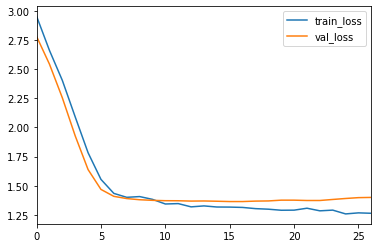After termination, the EarlyStopping callback loads the best performing model (in terms of validation loss). We can verify this by comparing the minimum validation loss to the validation score of the trained model.

In :
log.to_pandas().val_loss.min()

Out:
1.3641669750213623
In :
model.score_in_batches(val)

Out:
{'loss': 1.3641669750213623}

## Prediction¶

For evaluation we first need to obtain survival estimates for the test set. This can be done with model.predict_surv which returns an array of survival estimates, or with model.predict_surv_df which returns the survival estimates as a dataframe.

In :
surv = model.predict_surv_df(x_test)


We can plot the survival estimates for the first 5 individuals. Note that the time scale is correct because we have set model.duration_index to be the grid points. We have, however, only defined the survival estimates at the 10 times in our discretization grid, so, the survival estimates is a step function

In :
surv.iloc[:, :5].plot(drawstyle='steps-post')
plt.ylabel('S(t | x)')
_ = plt.xlabel('Time')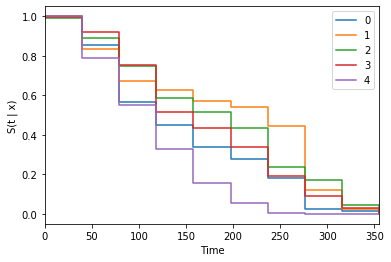It is, therefore, often beneficial to interpolate the survival estimates. Linear interpolation (constant density interpolation) can be performed with the interpolate method. We also need to choose how many points we want to replace each grid point with. Her we will use 10.

In :
surv = model.interpolate(10).predict_surv_df(x_test)

In :
surv.iloc[:, :5].plot(drawstyle='steps-post')
plt.ylabel('S(t | x)')
_ = plt.xlabel('Time')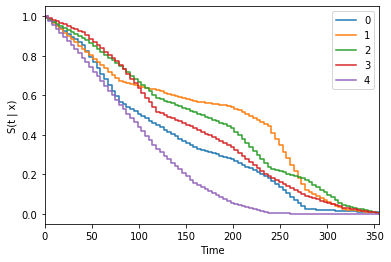## Evaluation¶

The EvalSurv class contains some useful evaluation criteria for time-to-event prediction. We set censor_surv = 'km' to state that we want to use Kaplan-Meier for estimating the censoring distribution.

In :
ev = EvalSurv(surv, durations_test, events_test, censor_surv='km')


#### Concordance¶

In :
ev.concordance_td('antolini')

Out:
0.6579549790156429

#### Brier Score¶

We can plot the the IPCW Brier score for a given set of times. Here we just use 100 time-points between the min and max duration in the test set. Note that the score becomes unstable for the highest times. It is therefore common to disregard the rightmost part of the graph.

In :
time_grid = np.linspace(durations_test.min(), durations_test.max(), 100)
ev.brier_score(time_grid).plot()
plt.ylabel('Brier score')
_ = plt.xlabel('Time')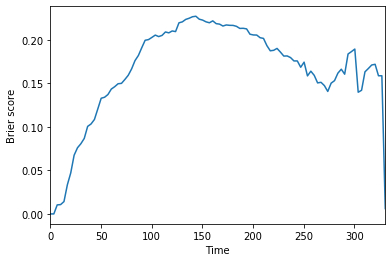#### Negative binomial log-likelihood¶

In a similar manner, we can plot the the IPCW negative binomial log-likelihood.

In :
ev.nbll(time_grid).plot()
plt.ylabel('NBLL')
_ = plt.xlabel('Time')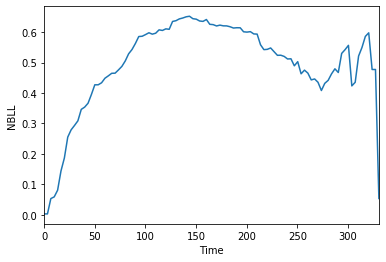#### Integrated scores¶

The two time-dependent scores above can be integrated over time to produce a single score Graf et al. 1999. In practice this is done by numerical integration over a defined time_grid.

In :
ev.integrated_brier_score(time_grid)

Out:
0.16609613377948
In :
ev.integrated_nbll(time_grid)

Out:
0.495728257223814

# Next¶

You can now look at other examples of survival methods in the examples folder. Or, alternatively take a look at

In [ ]: